Updating search results...

# 15 Results

View
Selected filters:
• UT.MATH.III.F.IF.4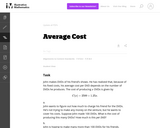Unrestricted Use
CC BY
Rating
0.0 stars

In this real world problem students solve questions based on the relationship between production costs and price.

Subject:
Mathematics
Secondary Mathematics
Material Type:
Activity/Lab
Provider:
Illustrative Mathematics
Provider Set:
Illustrative Mathematics
Author:
Illustrative Mathematics
05/01/2012Unrestricted Use
CC BY
Rating
0.0 stars

The purpose of this task is to give students practice constructing functions that represent a quantity of interest in a context, and then interpreting features of the function in the light of that context. It can be used as either an assessment or a teaching task.

Subject:
Mathematics
Secondary Mathematics
Material Type:
Activity/Lab
Provider:
Illustrative Mathematics
Provider Set:
Illustrative Mathematics
Author:
Illustrative Mathematics
05/01/2012Unrestricted Use
CC BY
Rating
0.0 stars

The primary purpose of this task is to lead students to a numerical and graphical understanding of the behavior of a rational function near a vertical asymptote, in terms of the expression defining the function. The canoe context focuses attention on the variables as numbers, rather than as abstract symbols.

Subject:
Mathematics
Secondary Mathematics
Material Type:
Activity/Lab
Provider:
Illustrative Mathematics
Provider Set:
Illustrative Mathematics
Author:
Illustrative Mathematics
05/01/2012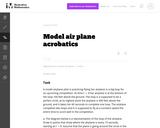Unrestricted Use
CC BY
Rating
0.0 stars

This is a task from the Illustrative Mathematics website that is one part of a complete illustration of the standard to which it is aligned. Each task has at least one solution and some commentary that addresses important asects of the task and its potential use. Here are the first few lines of the commentary for this task: A model airplane pilot is practicing flying her airplane in a big loop for an upcoming competition. At time $t=0$ her airplane is at the bottom of the ...

Subject:
Mathematics
Secondary Mathematics
Material Type:
Activity/Lab
Provider:
Illustrative Mathematics
Provider Set:
Illustrative Mathematics
Author:
Illustrative Mathematics
05/30/2013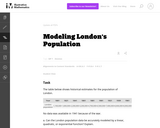Unrestricted Use
CC BY
Rating
0.0 stars

This is a task from the Illustrative Mathematics website that is one part of a complete illustration of the standard to which it is aligned. Each task has at least one solution and some commentary that addresses important asects of the task and its potential use. Here are the first few lines of the commentary for this task: The table below shows historical estimates for the population of London. Year18011821 18411861 18811901 1921 1939 1961 London population 1,100,000 1,60...

Subject:
Mathematics
Secondary Mathematics
Material Type:
Activity/Lab
Provider:
Illustrative Mathematics
Provider Set:
Illustrative Mathematics
Author:
Illustrative Mathematics
10/30/2013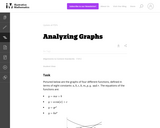Unrestricted Use
CC BY
Rating
0.0 stars

This is a task from the Illustrative Mathematics website that is one part of a complete illustration of the standard to which it is aligned. Each task has at least one solution and some commentary that addresses important asects of the task and its potential use. Here are the first few lines of the commentary for this task: Pictured below are the graphs of four different functions, defined in terms of eight constants: $a, b, c, k, m, p, q, \text{ and } r.$ The equations of...

Subject:
Mathematics
Material Type:
Activity/Lab
Provider:
Illustrative Mathematics
Provider Set:
Illustrative Mathematics
Author:
Illustrative Mathematics
08/20/2013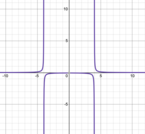Conditional Remix & Share Permitted
CC BY-NC
Rating
0.0 stars

This lesson includes a video&nbsp;lesson I did with my Secondary 3 Honors class that is a pre-lesson to graphing rational functions. In this video, we identify the horizontal and vertical asymptotes, holes, domain and range, and the x and y intercepts of rational functions.&nbsp; There is guided notes, a homework assignment, and a bell quiz also attached.

Subject:
Secondary Mathematics
Material Type:
Lesson
Author:
Tyrani Bevell
10/27/2021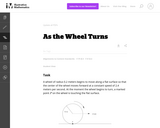Unrestricted Use
CC BY
Rating
0.0 stars

In this task, students use trigonometric functions to model the movement of a point around a wheel and, in the case of part (c), through space (F-TF.5). Students also interpret features of graphs in terms of the given real-world context (F-IF.4).

Subject:
Mathematics
Secondary Mathematics
Material Type:
Activity/Lab
Provider:
Illustrative Mathematics
Provider Set:
Illustrative Mathematics
Author:
Illustrative Mathematics
05/01/2012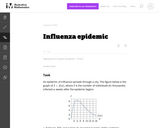Unrestricted Use
CC BY
Rating
0.0 stars

The purpose of this task is to probe students' ability to correlate symbolic statements about a function using function notation with a graph of the function, and to interpret their answers in terms of the quantities between which the function describes a relationship

Subject:
Mathematics
Secondary Mathematics
Material Type:
Activity/Lab
Provider:
Illustrative Mathematics
Provider Set:
Illustrative Mathematics
Author:
Illustrative Mathematics
05/01/2012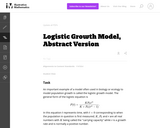Unrestricted Use
CC BY
Rating
0.0 stars

The goal of this task is to have students appreciate how the different constants (P0, K, and r) influence the shape of the graph.

Subject:
Mathematics
Secondary Mathematics
Material Type:
Activity/Lab
Provider:
Illustrative Mathematics
Provider Set:
Illustrative Mathematics
Author:
Illustrative Mathematics
08/15/2012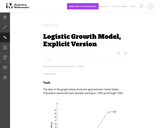Unrestricted Use
CC BY
Rating
0.0 stars

This problem introduces a logistic growth model in the concrete setting of estimating the population of the U.S. The model gives a surprisingly accurate estimate and this should be contrasted with linear and exponential models, studied in U.S. Population 1790-1860.'' This task requires students to interpret data presented.

Subject:
Mathematics
Secondary Mathematics
Material Type:
Activity/Lab
Provider:
Illustrative Mathematics
Provider Set:
Illustrative Mathematics
Author:
Illustrative Mathematics
08/15/2012Unrestricted Use
CC BY
Rating
0.0 stars

In this task students are given graphs of quantities related to weather. The purpose of the task is to show that graphs are more than a collection of coordinate points, that they can tell a story about the variables that are involved and together they can paint a very complete picture of a situation, in this case the weather.

Subject:
Mathematics
Secondary Mathematics
Material Type:
Activity/Lab
Provider:
Illustrative Mathematics
Provider Set:
Illustrative Mathematics
Author:
Illustrative Mathematics
05/01/2012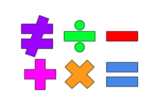Unrestricted Use
Public Domain
Rating
0.0 stars

This lesson is for a math classroom, but can be adapted to fit any grade, subject, or content.&nbsp;&nbsp;In this lesson, students will use an iPad and its features: Keynote, Pages, Garageband, Numbers, Presentation, and iMovie.&nbsp; Students will use 3 of those features/programs to create a video lesson consisting of several examples from a topic of their choice.&nbsp; This project is in place of a term final, so their chosen topic should be from their current term.Image citation: The image is one I created.&nbsp;&nbsp;

Subject:
Secondary Mathematics
Material Type:
Assessment
Author:
Tyrani Bevell
02/26/2022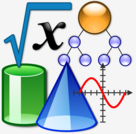Conditional Remix & Share Permitted
CC BY-NC
Rating
0.0 stars

Thumbnail photo:&nbsp;&nbsp;&quot;File:Nuvola Math and Inf.svg&quot;&nbsp;by&nbsp;Vojtěch Pikal&nbsp;is marked with&nbsp;CC0 1.0&nbsp;&nbsp;This is a lesson plan that can be used in any class and for any subject.&nbsp; The idea is to have the students review the content learned over the term, pick one of the concepts, and create a mini video lesson on the chosen concept.&nbsp; This is a project in place of a term final, but can be used at any time!I have tagged only a few math standards because this lesson applies to all content and all standards.

Subject:
Other
Material Type:
Lesson
Author:
Tyrani Bevell
12/03/2021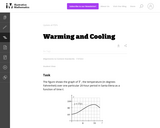Unrestricted Use
CC BY
Rating
0.0 stars

This task is meant to be a straight-forward assessment task of graph reading and interpreting skills. This task helps reinforce the idea that when a variable represents time, t=0 is chosen as an arbitrary point in time and positive times are interpreted as times that happen after that.

Subject:
Mathematics
Secondary Mathematics
Material Type:
Activity/Lab
Provider:
Illustrative Mathematics
Provider Set:
Illustrative Mathematics
Author:
Illustrative Mathematics